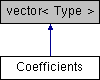tlx
PolynomialRegression< Type, WithStore >::Coefficients Struct Reference

polynomial stored as the coefficients of $$a_0+a_1 x^1+a_2 x^2+\cdots+a_n x^n$$ More...

#include <polynomial_regression.hpp>

Inheritance diagram for PolynomialRegression< Type, WithStore >::Coefficients:## Public Member Functions

Type evaluate (const Type &x) const
evaluate polynomial at x using Horner schema More...

## Detailed Description

### template<typename Type = double, bool WithStore = false> struct tlx::PolynomialRegression< Type, WithStore >::Coefficients

polynomial stored as the coefficients of $$a_0+a_1 x^1+a_2 x^2+\cdots+a_n x^n$$

Definition at line 84 of file polynomial_regression.hpp.

## Member Function Documentation

 Type evaluate ( const Type & x ) const
inline

evaluate polynomial at x using Horner schema

Definition at line 86 of file polynomial_regression.hpp.

The documentation for this struct was generated from the following file: